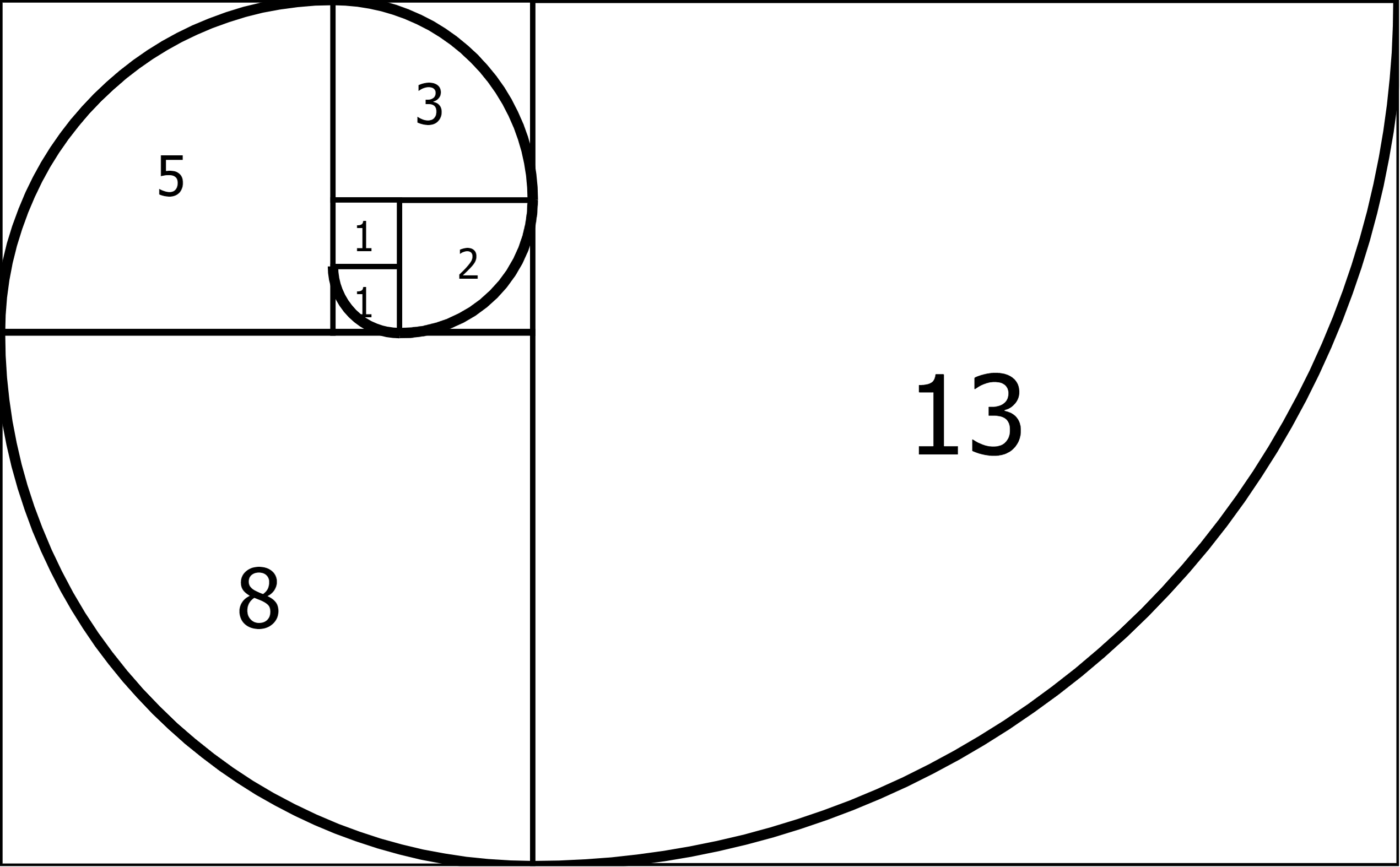# Fibonacci sequence

Fibonacci sequence and its usage. Fibonacci sequence in relationship to the Golden ratio. Weber's law estimations using the sequence.

## Fibonacci sequence

• Fn = Fn-1 + Fn-2 .
• Each number is the sum of the two preceding ones, starting from 0 and 1.
• Is ubiquitous: the tree branches, cauliflower and the curves of the human brain, etc. It’s a phenomena surrounding us everywhere.

### Weber’s law

• The difference we can identify between objects is given by a percentage.
• For example, if 60% difference can be distinguished in an effort between two estimates, we can distinguish that same percentage difference between other estimates and that is corresponding with the Fibonacci Sequence.
• Fibonacci values work well because they increase by about the same portion each time.

### Golden ratio

• Golden mean or golden section.
• Two quantities are in the golden ratio if their ratio is the same as the ratio of their sum to the larger of the two quantities.
• Any two successive Fibonacci Numbers, have ratio very close to the Golden Ratio “φ” which is approximately 1,618034…###### Resources
wikipedia.org mathsisfun.com wikipedia.org mathsisfun.com mountaingoatsoftware.com productplan.com Jeff Sutherland: Scrum - the art of doing twice the work in half the time